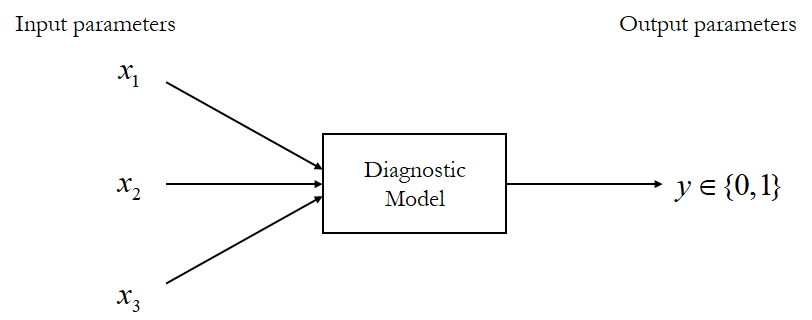In clinical statistics, many people know that the exponential of logistic regression coefficients makes the odds ratio. However, there is a very simple and straightforward explanation to that which is omitted by most online blog posts of this topic. I am here to do the extremely simple math to show you why.

## Measurement of altered probability

Now we confine our discussion in a diagnostic model, which means the output of the model has a binary explanation: positive or negative, 0 or 1.Let us say this model predicts the status of disease $y\in {0,1}$, based on one or more input variables $x_i$ (often called explanatory variables). Although a bivariate assertion have to be made, there is a probability of having the disease $\pi$, for any fixed combination of explanatory variables.

In a diagnostic model, we often want to know (or measure) the impact of some explanatory variable on the final probability $\pi$. Suppose there is a baseline state as a reference, where we have a probability of $\pi_0$. Then, the variable changed, leading to an altered probability of $\pi_1$. So the straightforward way to measure the change would be the relative risk ($\mathrm{RR}$):

$\mathrm{RR} = \frac{\pi_1}{\pi_0}$

Odds, the probability of something happen over the probability of that thing not happen, is just a weird way to express the likelihood. The odds of something with a probability of $\pi$ is defined as:

$\mathrm{Odds} = \frac{\pi}{1-\pi}$

So, in that case, the odds ratio (OR) is simply defined as the ratio of the two odds:

$\mathrm{OR} = \frac{\mathrm{Odds_1}}{\mathrm{Odds_0}} = \frac{\frac{\pi_1}{1-\pi_1}}{\frac{\pi_0}{1-\pi_0}}$

## The logistic regression

I suppose everyone knows the logistic regression, as it is a diagnostic model to predict a probability of having a disease $p$, based on some explanatory variables $x_i$. The model assumes that the explanatory variables have an linear relationship with the logit of $p$, where

$\mathrm{logit}(p) = \log(\mathrm{Odds}) = \log(\frac{p}{1-p})$

Naturally, the form of logistic regression is,

$\log(\frac{p}{1-p}) = \sum_i \beta_ix_i+\beta_0$

I dropped the error term for simplicity.

After this, a cut-off value $C$ is determined, and any $p$ greater than $C$ is considered a positive prediction, and vice versa.

## Finding the odds ratio

Let us first suppose a case where we have three explanatory variables, $x_1$, $x_2$, and $x_3$. Here, $x_1$ is a discrete variable which has two states, 0 or 1. We want to know the effect of $x_1$ on the outcome. So, a logistic regression was done, and a logistic model was obtained:

$\log(\frac{p}{1-p}) = \beta_0 + \beta_1x_1 + \beta_2x_2 + \beta_3x_3$

Now when $x_1$ is 0, and $x_2$ and $x_3$ are some random but fixed value $X_2$ and $X_3$, we can obtain a baseline probability $p_0$.

$\log(\frac{p_0}{1-p_0}) = \beta_0 + \beta_2X_2 + \beta_3X_3$

No hurry to calculate $p_0$ (although it is very easy), we now get the logit of probability $p_1$ when $x_1$ is 1.

$\log(\frac{p_1}{1-p_1}) = \beta_0 + \beta_1 + \beta_2X_2 + \beta_3X_3$

So how about the odds ratio with respect of $x_1$? We are going to do a subtraction with the two equations above.

\begin{aligned} \log(\frac{p_1}{1-p_1}) - \log(\frac{p_0}{1-p_0}) =& (\beta_0 + \beta_1 + \beta_2X_2 + \beta_3X_3) - \\ &(\beta_0 + \beta_2X_2 + \beta_3X_3)\\ \log(\frac{\mathrm{Odds_1}}{\mathrm{Odds_0}})=& \beta_1\\ \log(\mathrm{OR}) =& \beta_1\\ \mathrm{OR} =& \exp(\beta_1) \end{aligned}

So, the OR associated with the factor $x_1$ is simply $\exp(\beta_1)$. The confidence interval and the hypothesis test $P$-value of the OR can be calculated accordingly.

## Remarks

• The OR calculated has been adjusted by the other two factors ($x_2$ and $x_3$).

It is obvious, but still worth mentioning. If the unadjusted OR is desired (I really doubt if it is ever required), you have got to build a logistic model with only one explanatory variable $x_1$.

• The above reasoning applies to bivariate explanatory variables.

For continuous variables, the OR is somewhat analogy. What really happen for variable $x_j$ is

$\frac{\partial \log(\mathrm{Odds})}{\partial x_j} = \beta_j$

given a $\Delta x_j = 1$, we can get the OR with $x_j$ changing by 1.

$\mathrm{OR}_j = \exp(\beta_j)$
• Use the oddsratio package in R. Its document is written very well.